# SRMJEEE Maths Mock Test - 4

## 35 Questions MCQ Test SRMJEEE Subject Wise & Full Length Mock Tests | SRMJEEE Maths Mock Test - 4

Description
Attempt SRMJEEE Maths Mock Test - 4 | 35 questions in 50 minutes | Mock test for JEE preparation | Free important questions MCQ to study SRMJEEE Subject Wise & Full Length Mock Tests for JEE Exam | Download free PDF with solutions
QUESTION: 1

Solution:
QUESTION: 2

Solution:
QUESTION: 3

### If r and n are positive integers ; r > 1, n > 2 and coefficient of (r + 2)th term and (3r)th term in the expansion of (1 + x)2n are equal, then n equals

Solution:
QUESTION: 4

Let [ ] denote the greatest integer function and f(x) = [tan2x]. Then

Solution: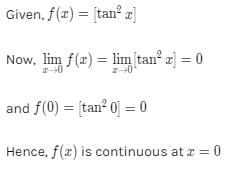QUESTION: 5

If ω is non-real cube root of unity then

[(1 + 2ω + 3ω2) /(2 + 3ω + ω2)] + [(2 + 3ω + 3ω2)/(3 + 3ω + 2ω2)] is equal to

Solution:
QUESTION: 6

The volume of a solid which is obtained by revolving area bounded by an ellipse x2+9y2=9 and straight line x+3y=3 about y-axis is

Solution:
QUESTION: 7

The equation x2 + y2 + x + y + 1 = 0 represents a circle whose centre is at

Solution:
QUESTION: 8

The coordinates of three vertices of parallelogram are (1,3)(2,0) and (5,1), then its 4th vertex is

Solution:
QUESTION: 9

The eccentricity of parabola is

Solution:

Parabola is the locus of a point, say P, which moves such that its distance from a fixed point, say S, is equal to its distance from a fixed line say l.

Eccentricity is defined as the ratio of the distance of the moving point P from the fixed point S, to its distance from a fixed line l.

It is denoted by e. Draw PM perpendicular to l. Then, eccentricity e = PS/PM

Since the two distances are equal in case of a parabola, PS = PM. So, PS/PM = 1.

Therefore, we say eccentricity of a parabola is 1.

In case of an ellipse it is less than 1 and in case of a hyperbola it is greater than 1.

QUESTION: 10

The roots of the equation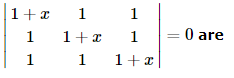Solution: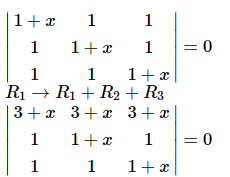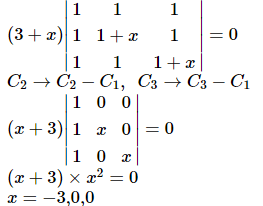QUESTION: 11

If x dy = y(dx + y dy), y > 0 and y (1) = 1, then y (-3) is equal to

Solution:
QUESTION: 12

sec2(tan⁻1 2) + cosec2(cot⁻1 3) =

Solution:
QUESTION: 13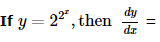Solution:
QUESTION: 14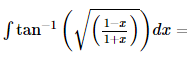Solution:
QUESTION: 15

What is the value of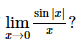Solution:
QUESTION: 16

If A and B are non-singular matrix, then

Solution:
QUESTION: 17

The inverse of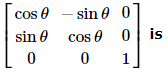Solution:
QUESTION: 18

The strength of a beam varies as the product of its breadth b and square of its depth d. A beam cut out of a circular log of radius r would be strong when

Solution:

Strength of the beam S = kbd2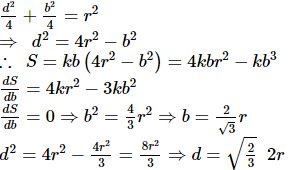QUESTION: 19

The mode of the following observation is 10,8,-9,-12,15,0,23,-3,-2,-13,-24,28,35,42

Solution:
QUESTION: 20

The S.D. of a distribution is 5. The value of the fourth central moment μ4 , in order that the distribution be mesokurtic should be

Solution:
QUESTION: 21

If nPr=840, nCr=35, then n=

Solution: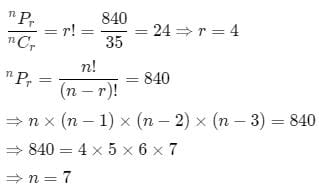QUESTION: 22

A coin is tossed 10 times. The probability of getting exactly six heads, is

Solution:
QUESTION: 23

The base of triangle is passing through a fixed point (a,b) and its sides are bisected at right angles by lines, y2 - 4xy - 5x2 = 0, then the locus of the vertex of triangle is

Solution: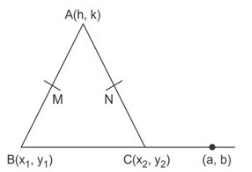y2 - 4xy - 5x2 = 0
⇒ (y - 5x) (y + x) = 0
y - 5x, cuts AB at M at right angle
and y + x = 0 cuts AC at N at right angle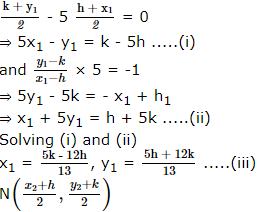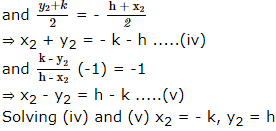By collinearity of (x1, y1), (x2, y2), (a, b) we get h = x, k = y
2x2 + 2y2 + (3a + 2b)x + (2a - 3b)y = 0

QUESTION: 24

In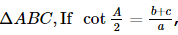, then the triangle is

Solution:
QUESTION: 25

If the roots of the equation ax2 + bx + c = 0 are l and 2l, then

Solution:
QUESTION: 26

1 + (3/2) + (5/22) + (7/23) + ... to ∞ is equal to

Solution:
QUESTION: 27

In Rule Method the null set is represented by

Solution:
QUESTION: 28

The derivative of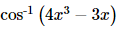with respect to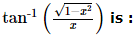Solution:
QUESTION: 29

The equation of line joining points (-5,-6) and (3,10) is

Solution:
QUESTION: 30

P(2,2) and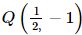are two points on the parabola y2 =2x. The coordinates of the point R on the parabola, where the tangent to the curve is parallel to the chord PQ, is

Solution: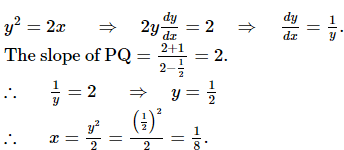QUESTION: 31

The solution set of (2cosx-1) (3+2cosx) = 0 in the interval 0≤x≤2π is

Solution:
QUESTION: 32

1+cos2x+cos4x+cos6x=

Solution:
QUESTION: 33

The area of a parallelogram is 5√3, its diagonals are

Solution:
QUESTION: 34

The sine of the angle between the vectors î - 2 ĵ + 3 k̂ and 2 î + ĵ + k̂ is

Solution:
QUESTION: 35

If 3 is the mean and 3/2 is the S.D. of a binomial distribution, then distribution is

Solution:Use Code STAYHOME200 and get INR 200 additional OFF Use Coupon Code